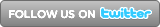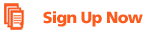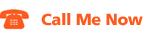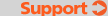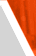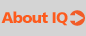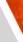homesite mapcontact us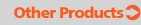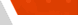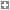Contact UsFAQsDownloadSymbol GuideForum

## The "Greeks" Defined

The delta, theta, gamma, rho and vega parameters within an Options Chain measure option price sensitivity to changes in the price of the underlying stock, time to the option's expiration, prevalent interest rates and underlying stock price volatility.Delta. Mathematically, delta is defined as the first partial derivative of the option price with respect to the underlying stock price, and is a measure of the option price sensitivity to changes of the underlying stock price. The gain (loss) of the option price roughly equals to delta times increase (decrease) of the stock price provided that other parameters (time to expiration, interest rate and volatility) are fixed.

Theta. Mathematically, theta is defined as the first partial derivative of the option price with respect to the time to expiration and is a measure of the option price sensitivity to the passage of time. The expected gain (loss) of the option price during a certain period of time roughly equals to theta times this period provided that during that time other parameters (stock price, interest rate and volatility) are fixed.

Rho. Mathematically, rho is defined as the first partial derivative of the option price with respect to the interest rates and is a measure of the option price sensitivity to changes of this parameter. The expected gain (loss) of the option price roughly equals to rho times the increase (decrease) in interest rate provided that other parameters (stock price, time to expiration and volatility) are fixed.

Vega. Mathematically, vega is defined as the first partial derivative of the option price with respect to volatility and is a measure of the option price sensitivity to changes of this parameter. The expected gain (loss) of the option price roughly equals to vega times the increase (decrease) of volatility provided that other parameters (stock price, time to expiration and interest rates) are fixed.

Gamma. Mathematically, gamma is defined as the first partial derivative of delta (i.e. the second partial derivative of the option price) with respect to the underlying stock price, and in a measure of the delta sensitivity to changes of the underlying stock price. The gain (loss) of the option's delta roughly equals to gamma times increase (decrease) of the stock price. Gamma is normally used in relatively complicated trading strategies.

 Powered by FinWin.com © 2022 DTN. All rights reserved. All Equities and Futures data is delayed according to exchange rules. NYSE and AMEX at least 20 minutes. Nasdaq at least 15 minutes. CME and CBOT at least 10 minutes. NYBOT, NYMEX and COMEX at least 30 minutes.

[Form: 328]Have a Question for Sales?
Call 800-475-4755
or
Email sales@dtniq.com